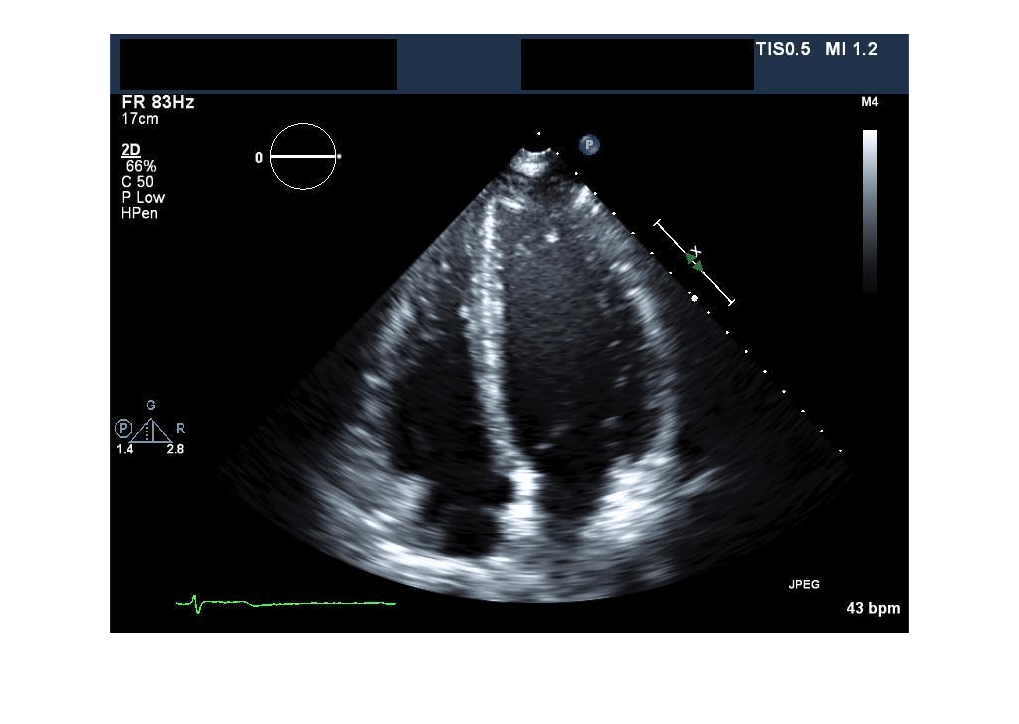# extractFrame

Extract pixel data for one frame of 2-D medical image series

## Syntax

``X = extractFrame(medImage,frame)``

## Description

example

````X = extractFrame(medImage,frame)` extracts the pixel data for the frame at index `frame` of the `medicalImage` object `medImage`.```

## Examples

collapse all

Specify the name of an echocardiogram series stored as a DICOM file.

`fileName = "heartUltrasoundSequence.dcm";`

Create a medical image object for the echocardiogram series.

`medImage = medicalImage(fileName);`

Check the `NumFrames` property value to determine the number of frames in the series. `medImage` contains three frames.

`numFrames = medImage.NumFrames`
```numFrames = 3 ```

Extract the second frame in the image series. Display the extracted frame image.

```X = extractFrame(medImage,2); imshow(X)```## Input Arguments

collapse all

Medical image, specified as a `medicalImage` object.

Frame index, specified as a positive integer scalar in the range [1, numFrames], where numFrames is the number of frames in the image or series of images in `medImage`. The number of frames is specified by the `NumFrames` property of `medImage`.

Data Types: `double`

## Output Arguments

collapse all

Pixel data of the extracted frame, returned as an m-by-n numeric matrix, where m and n are the first two dimensions of the image frame extracted from `medImage`. The `X` output matrix is the same data type as the `Pixels` property of `medImage`.

## Version History

Introduced in R2022b